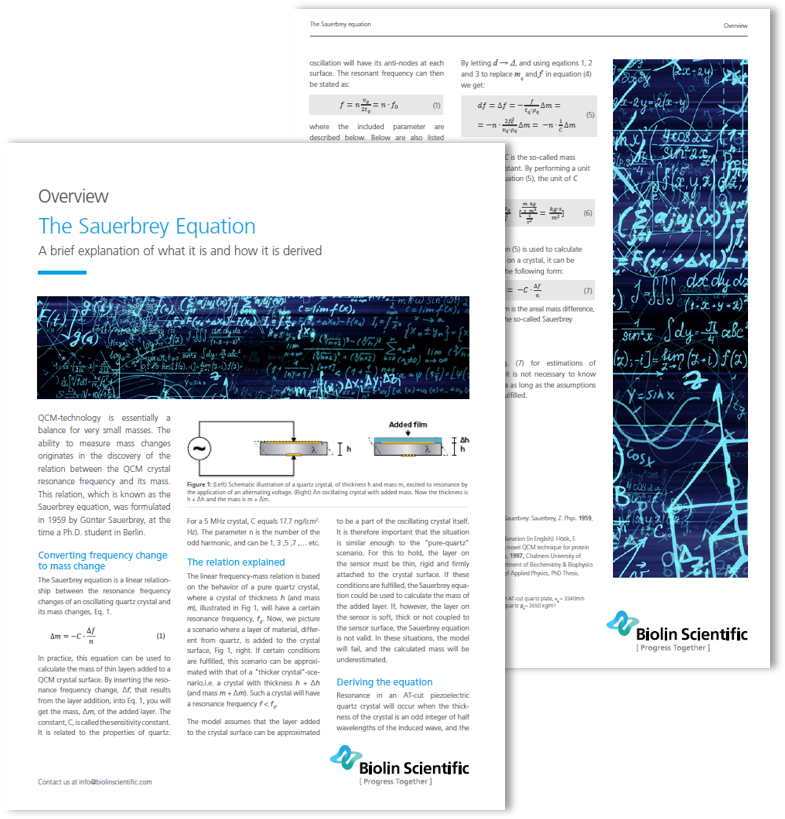# The Sauerbrey relation

- how it is derived and what the parameters mean

In the context of QCM technology, there is often a reference to the so-called Sauerbrey equation. The Sauerbrey equation is a linear relationship between the resonance frequency of an oscillating quartz crystal and mass changes. It can be used to calculate the mass of thin and rigid films deposited on the sensor.

In this overview we derive the equation and explain the different parameters.### Fill out the form to access the overview